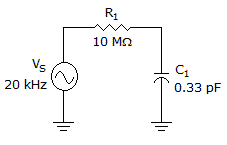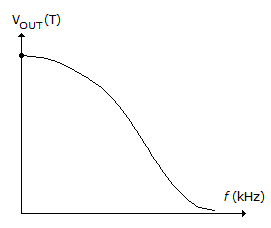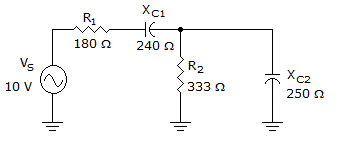# Electronics - RC Circuits

Exercise : RC Circuits - General Questions
6.Calculate the magnitude of the impedance in the given circuit.

24.1 M10 M26.1 M0Explanation:
No answer description is available. Let's discuss.

7.Calculate the phase angle in the given circuit.

90°
22.52°
67.48°
Explanation:
No answer description is available. Let's discuss.

8.
What is the phase angle for a parallel circuit consisting of a 500 kHz, 5 Vac source with a 47 pF capacitor, and a 4.7 kresistor in parallel?
55.3°
–55.3°
34.8°
–34.8°
Explanation:
No answer description is available. Let's discuss.

9.Which circuit is represented by the frequency response curve in the given figure?

High-pass filter
Low-pass filter
Band-pass filter
Band-stop filter

Explanation:
No answer description is available. Let's discuss.

10.What is the phase angle in the given circuit?

14.95°
36.88°
90°